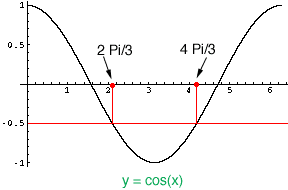Quandaries and Queries go to http://www.nysedregents.org/testing/mathre/mathbaug04.pdf then question#33 I solved for cosine and got cos(pi/6 t)<=1/2, and then cos(30 degrees * t)<=1/2, but then I couldn't go further to solve for t.Please help.Thank you. Hi Abraham, The problem states The tide at a boat dock can be modeled by the equation y = -2cos(pi/6 t) + 8, where t is the number of hours past noon and y is the height of the tide, in feet. For how many hours between t=0 and t=12 is the tide at least 7 feet? I solved for the cosine when the tide is 7 feet and got 7 = -2cos(pi/6 t) + 8, so cos(pi/6 t) = -1/2 I then sketched y = cos(x) for x between 0 and 2 Pi radians.cos(x) = -1/2 when x = 2 Pi/3 radians and at x = 4 Pi/3 radians. Thus cos(pi/6 t) is less than -1/2 for Pi/6 t between 2 Pi/3 and 4 Pi/3. Pi/6 t = 2 Pi/3 gives t = 4 hours, and Pi/6 t = 4  Pi/3 gives t = 8 hours. Thus the tide is less than 7 feet for the 4 hours between 4 and 8, and hence the tide is at least 7 feet for 8 hours. Penny Go to Math Central